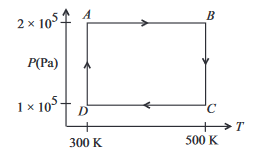# Two moles of helium gas are taken over the cycle ABCDA, as shown in the P - T diagram  Assuming the gas to be ideal the work done on the ga...

Two moles of helium gas are taken over the cycle ABCDA, as shown in the P - T diagramAssuming the gas to be ideal the work done on the gas in taking it from A to B is

Anonymous User Physics Thermodynamics 09 May, 2020 54 views